# Search by Topic

#### Resources tagged with Number - generally similar to N000ughty Thoughts:

Filter by: Content type:
Age range:
Challenge level:

### There are 30 results

Broad Topics > Numbers and the Number System > Number - generally### N000ughty Thoughts

##### Age 14 to 16 Challenge Level:

How many noughts are at the end of these giant numbers?### Mod 3

##### Age 14 to 16 Challenge Level:

Prove that if a^2+b^2 is a multiple of 3 then both a and b are multiples of 3.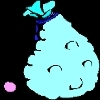### Inequalities

##### Age 11 to 14 Challenge Level:

A bag contains 12 marbles. There are more red than green but green and blue together exceed the reds. The total of yellow and green marbles is more than the total of red and blue. How many of. . . .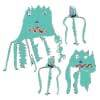### Back to the Planet of Vuvv

##### Age 11 to 14 Challenge Level:

There are two forms of counting on Vuvv - Zios count in base 3 and Zepts count in base 7. One day four of these creatures, two Zios and two Zepts, sat on the summit of a hill to count the legs of. . . .### Cross-country Race

##### Age 11 to 14 Challenge Level:

Eight children enter the autumn cross-country race at school. How many possible ways could they come in at first, second and third places?### Find the Fake

##### Age 14 to 16 Challenge Level:

There are 12 identical looking coins, one of which is a fake. The counterfeit coin is of a different weight to the rest. What is the minimum number of weighings needed to locate the fake coin?### Is it Magic or Is it Maths?

##### Age 11 to 14 Challenge Level:

Here are three 'tricks' to amaze your friends. But the really clever trick is explaining to them why these 'tricks' are maths not magic. Like all good magicians, you should practice by trying. . . .### Euler's Officers

##### Age 14 to 16 Challenge Level:

How many different ways can you arrange the officers in a square?### Eleven

##### Age 11 to 14 Challenge Level:

Replace each letter with a digit to make this addition correct.### Factorial

##### Age 14 to 16 Challenge Level:

How many zeros are there at the end of the number which is the product of first hundred positive integers?### Multiplication Magic

##### Age 14 to 16 Challenge Level:

Given any 3 digit number you can use the given digits and name another number which is divisible by 37 (e.g. given 628 you say 628371 is divisible by 37 because you know that 6+3 = 2+7 = 8+1 = 9). . . .### Palindromes

##### Age 5 to 14

Find out about palindromic numbers by reading this article.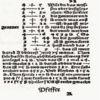### Mathematical Symbols

##### Age 5 to 14

A brief article written for pupils about mathematical symbols.### Mathematical Swimmer

##### Age 11 to 14 Challenge Level:

Twice a week I go swimming and swim the same number of lengths of the pool each time. As I swim, I count the lengths I've done so far, and make it into a fraction of the whole number of lengths I. . . .### Chocolate Maths

##### Age 11 to 14 Challenge Level:

Pick the number of times a week that you eat chocolate. This number must be more than one but less than ten. Multiply this number by 2. Add 5 (for Sunday). Multiply by 50... Can you explain why it. . . .### More Mods

##### Age 14 to 16 Challenge Level:

What is the units digit for the number 123^(456) ?### Card Trick 2

##### Age 11 to 14 Challenge Level:

Can you explain how this card trick works?### Calendar Capers

##### Age 11 to 14 Challenge Level:

Choose any three by three square of dates on a calendar page...### A Story about Absolutely Nothing

##### Age 7 to 18

This article for the young and old talks about the origins of our number system and the important role zero has to play in it.### Water Lilies

##### Age 11 to 14 Challenge Level:

There are some water lilies in a lake. The area that they cover doubles in size every day. After 17 days the whole lake is covered. How long did it take them to cover half the lake?### Latin Numbers

##### Age 14 to 16 Challenge Level:

Can you create a Latin Square from multiples of a six digit number?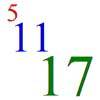### Ways of Summing Odd Numbers

##### Age 11 to 14

Sanjay Joshi, age 17, The Perse Boys School, Cambridge followed up the Madrass College class 2YP article with more thoughts on the problem of the number of ways of expressing an integer as the sum. . . .### An Investigation Based on Score

##### Age 11 to 14

Class 2YP from Madras College was inspired by the problem in NRICH to work out in how many ways the number 1999 could be expressed as the sum of 3 odd numbers, and this is their solution.### The Patent Solution

##### Age 11 to 14 Challenge Level:

A combination mechanism for a safe comprises thirty-two tumblers numbered from one to thirty-two in such a way that the numbers in each wheel total 132... Could you open the safe?### Pairs

##### Age 11 to 14 Challenge Level:

Ann thought of 5 numbers and told Bob all the sums that could be made by adding the numbers in pairs. The list of sums is 6, 7, 8, 8, 9, 9, 10,10, 11, 12. Help Bob to find out which numbers Ann was. . . .### All Is Number

##### Age 7 to 14

Read all about Pythagoras' mathematical discoveries in this article written for students.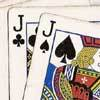### Do Unto Caesar

##### Age 11 to 14 Challenge Level:

At the beginning of the night three poker players; Alan, Bernie and Craig had money in the ratios 7 : 6 : 5. At the end of the night the ratio was 6 : 5 : 4. One of them won \$1 200. What were the. . . .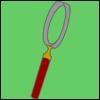### The Secret World of Codes and Code Breaking

##### Age 7 to 16

When you think of spies and secret agents, you probably wouldn’t think of mathematics. Some of the most famous code breakers in history have been mathematicians.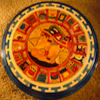### Calendars

##### Age 5 to 14

Calendars were one of the earliest calculating devices developed by civilizations. Find out about the Mayan calendar in this article.### Big and Small Numbers in Biology

##### Age 14 to 16 Challenge Level:

Work with numbers big and small to estimate and calulate various quantities in biological contexts.